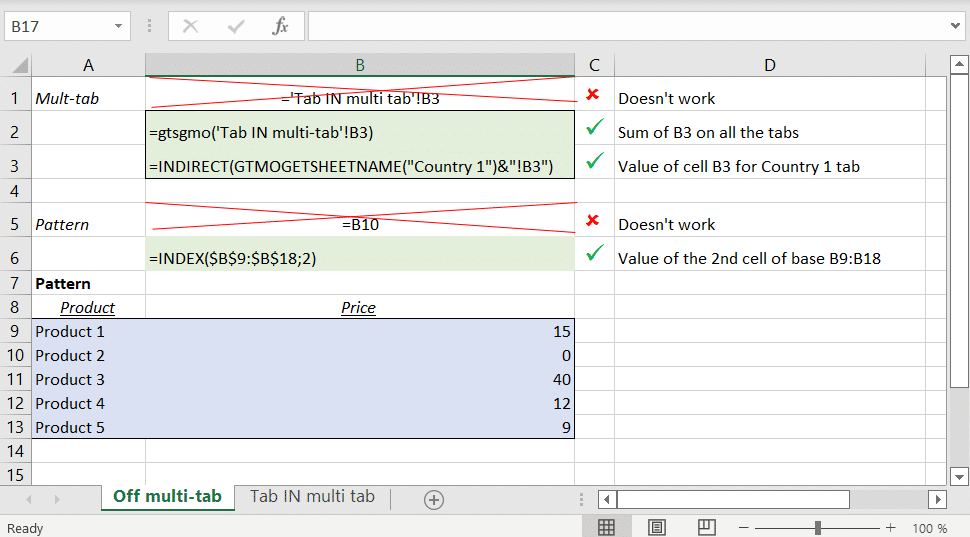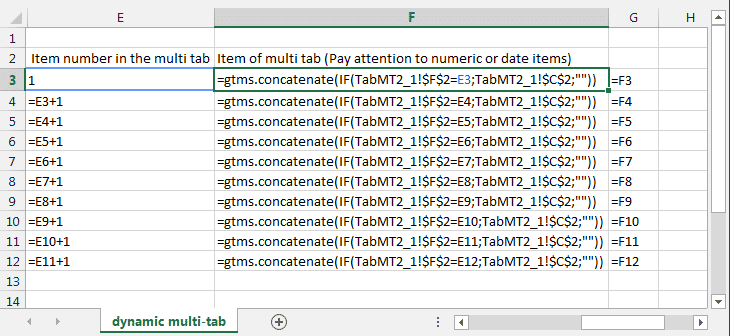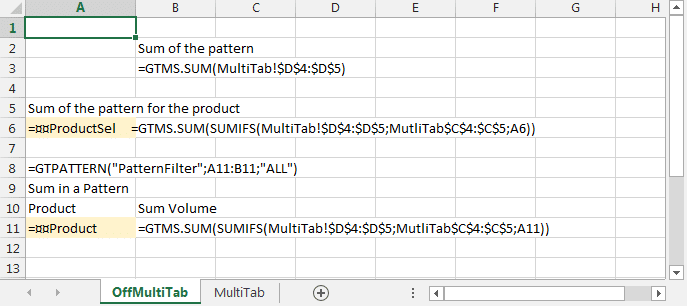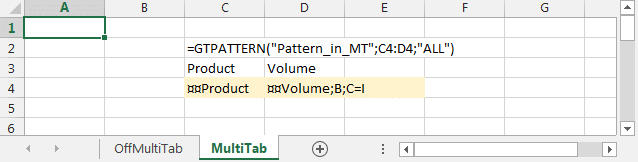# How can we help?

Search for answers or browse our knowledge base

# Multi-tab Data Synthesis

## General

For patterns and multi-tabs, it isn’t possible to construct a formula outside the pattern or multi-tab that points directly to a cell in the pattern or multi-tab.

In the case of a pattern, functions of access to tables (INDEX, MATCH, SEARCH, SUM, SUMIF etc…) may be used.

GTMS.xxxx functions generally have no equivalent in Excel for accessing ranges located “transversely” on tabs (3D references exist in Excel but they can only be used by a small number of functions).

This article gives application examples for the two main non-multi-tab data extraction techniques:

• use of GTMS.function_agreg(expression) which allows to sum the values of the same cell address on the tabs of the multi-tab.
• use of GTMOGETSHEETNAME with an INDIRECT allowing you to directly designate the tab and the address of the cell whose value you want to recover.

Note that these two functions can only be used in cells (not in conditional formatting, locks, etc.).A simple example is a table of contents listing the tabs with hyperlinks to each tab

The principle, used in most of the examples in this article, is to use a pattern listing the multi-tab items and to build hyperlinks to each of the tabs using GT functions.

An example is included in the file attached to this tutorial.

## Aggregations on tabs: GTMS.

The functions GTMS.xxxx allow data to be aggregated in the tabs of a multi-tab.

Functions GTMS. allowed :

```=GTMS.SUM(expression)
=GTMS.COUNT(expression)
=GTMS.COUNTA(expression)
=GTMS.MAX(expression)
=GTMS.MIN(expression)
=GTMS.AVERAGE(expression)
=GTMS.CONCATENATE(expression)
```

### Use

The expression used in the GTMS must contain at least one range to a multi-tab and must be one of the following types:

• GTMS.xxx(Range), as in
=GTMS.SUM(TabMT!\$B\$3)
• GTMS.xxx(function(formula)) as in
=GTMS.SUM(SUM(TabMT!\$A\$1 ; TabMT!\$A\$1:\$B\$3 ; OffTabMT!C12))

Ranges pointed to by expression cannot refer to several groups of multi-tabs.

GTMS functions are only allowed in formulas written in cells (not in conditional formulas, nor locks, nor validation constraints, etc.).

All references to tabs other than the current tab must be entered in absolute (\$A\$1).

### Mechanism and examples

The formula of the type

```=GTMS.SUM( TabMT!\$B\$3 )
```

will be dynamically replaced by an expression of the type

```=SUM(TabMT_1!\$B\$3;TabMT_2!\$B\$3;TabMT_3!\$B\$3)
```

The formula of the type

```=GTMS.SUM( IF( TabMT!\$B\$3>0 ; 0 ; 1) )
```

will be dynamically replaced by an expression of the type

```=SUM(IF(TabMT_1!\$B\$3>0;0;1) ; IF(TabMT_2!\$B\$3>0;0;1) ; IF(TabMT_3!\$B\$3>0;0;1) )
```

The formula of the type

```=GTMS.SUM(SUMIFS( TabMT!\$B\$3:\$B\$12 ; TabMT!\$A\$3:\$A\$12 ; OffTabMT!C15 ))
```

will be dynamically replaced by an expression of the type

```=SUM( SUMIFS(TabMT_1!\$B\$3:\$B\$12;TabMT_1!\$A\$3:\$A\$12;OffTabMT!C15) ;
SUMIFS(TabMT_2!\$B\$3:\$B\$12;TabMT_2!\$A\$3:\$A\$12;OffTabMT!C15);
SUMIFS(TabMT_3!\$B\$3:\$B\$12;TabMT_3!\$A\$3:\$A\$12;OffTabMT!C15))
```

This type of formula allows for example to make aggregations on the patterns located in multi-tabs.

The formula of the type =GTMS.SUM(TabMT!\$B\$3 + TabMT!\$A\$3) isn’t allowed.

An example is included in the file attached to this tutorial.

### restrictions

If an Excel export of the questionnaire is made, Excel will not support too many tabs used in the GTMS formulas.
Les limitations suivantes sont en cause :
– no more than 255 arguments in the Excel formulas: GTMS will generate an error in the Excel export if more than 255 tabs.
– formulas cannot exceed 8000 characters in Excel: the formula generated by the GTMS function will cause an error in the Excel export if it exceeds 8000 characters (including the size of the tab names used to reference the ranges, etc.)

Finally, when opening in Answer (and adding/removing tabs) if the multi-tab is dynamic, if a large number of GTMS formulas are used on a multi-tab group with a large number of tabs, Answer’s performance may be severely penalized.

For example, using more than 9,000 GTMS formulas pointing to a tab with more than 30 tabs will severely penalize opening performance in Answer.

A compromise must be found between the use of global aggregation functions such as GTMS and the use of INDIRECT functions associated with GTMOGETSHEETNAME or GTMOAXISVAL.

## Extraction of isolated values

### Extraction of isolated values with GTMS.SUM or GTMS.CONCATENATE

Consists in summing (respectively concatenate) a null value (resp. “”) on all the tabs except on the tab whose value is to be recovered

Considering a case where

• The cell TabMT!A2 of the multi-tab contains the multitab item
• The cell TabMT!B3 of the multi-tab contains the value to aggregate
• The cell OFF_MT!A3 contains the multi-tab item for which you want to extract the values

we can use

• for a numeric value or a date, the formula
=GTMS.SUM( IF(TabMT!\$B\$3 = OFF_MT!A3 ; TabMT!\$B\$3 ; 0))
• for a string, the formula
=GTMS.CONCATENATE( IF(TabMT!\$B\$3 = OFF_MT!A3 ; TabMT!\$B\$3 ; “”))

The advantage of this solution is that it doesn’t use INDIRECT functions that can have a significant negative impact on performance.

### Extraction of isolated values with INDIRECT and GTMOGETSHEETNAME

The INDIRECT function uses, as in Excel, the first argument, a string expression, to return the reference corresponding to this string (in the A1 syntax of Excel).

example =INDIRECT(“A”&”2”) returns the contents of cell A2.

The function GTMOGETSHEETNAME, specific to Answer, returns the name of the tab corresponding to a multi-tab item for a multi-tab axis.

By combining these two functions, a formula can access a cell in a tab of the multi-tab.

example: =INDIRECT(GTMOGETSHEETNAME(“Multi-Tab Item“)&”!A1″).As a reminder, it is best to isolate the GTMOGETSHEETNAME(“Multi-Tab Item”) function in a cell without any other function in the formula.

The INDIRECT function is a volatile function: it is systematically recalculated for any value modification in any cell of the workbook or any row/column addition).
The INDIRECT function (in Excel as in a GT questionnaire) should therefore only be used when there are no performance problems (due to data volumes or computational complexities) in the questionnaire.
Note that the use of INDIRECT if performed on a plain text reference (“hard”) may cause problems in the maintenance of the questionnaire design workbook: the addition of rows or columns before the addressed cell will not be taken into account by the plain text reference.

An example is included in the file attached to this tutorial.

## Extract the list of items from a dynamic multi-tab

This technique is explained in the ‘GTMS Synthesis 1′ tab of the GTTut-SynthGTMS-UK-Qst workbook (columns E and following).

It consists of :
– in the multi-tab, retrieve the index (or item number) of the current multi-tab item using the GTMOITEMNUM() function. In the example in F2
– off the multi-tab, build an index list (stopping at a maximum number) 1, 2, 3
outside the multi-tab, concatenate all multi-tab items (in the example, in C2 of the multi-tab) using the GTMS.CONCATENATE function, but returning the item to concatenate only when the cell containing GTMOITEMNUM() in the multi-tab tab tab is equal to the current index (outside the multi-tab)
The maximum number of items should be planned in advance (maximum 10 items in the example).

## Pattern synthesis in multi-tabsThe GTMS.xxx functions make it very easy to perform this type of operation. The expression contained in GTMS.xxx(expression) can point directly to the full range of a pattern (+ one line like the usual pattern operations).